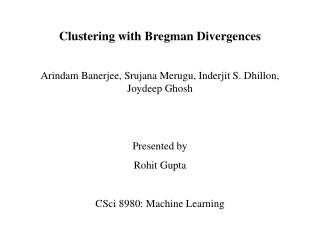DownloadDownload PresentationClustering with Bregman Divergences

# Clustering with Bregman Divergences

Télécharger la présentation## Clustering with Bregman Divergences

- - - - - - - - - - - - - - - - - - - - - - - - - - - E N D - - - - - - - - - - - - - - - - - - - - - - - - - - -
##### Presentation Transcript

1. Clustering with Bregman Divergences Arindam Banerjee, Srujana Merugu, Inderjit S. Dhillon, Joydeep Ghosh Presented by Rohit Gupta CSci 8980: Machine Learning

2. Outline • Bregman Divergences – Basics and Examples • Bregman Information • Bregman Hard Clustering • The Exponential Family and connection to Bregman Divergence • Bregman Soft Clustering • Experiments and Results • Conclusions

3. Bregman Hard and Soft Clustering • Most existing parametric clustering methods partition the data into pre-specified number of partitions with cluster representative corresponding to every partition/cluster • Hard Clustering – disjoint partitioning of the data such that each data point belongs to exactly one of the partitions • Soft Clustering – each data point has a certain probability of belonging to each of the partitions • Hard Clustering can be seen as Soft Clustering when probabilities are either 0 or 1

4. Distortion or Loss Functions • Squared euclidean distance is the most commonly used loss function • Extensive literature • Easy to use – leads to simple calculations • Not appropriate for some domains • Difficult to compute for sparse data (missing dimensions) • Example: Iterative K-means algorithm • Question: How to choose a distortion/loss function for a given problem?

5. Bregman Divergences • Ref: Definition 1 in the paper: • Examples: • Squared distance • Relative Entropy (KL divergence) • Itakura Saito distance

6. Few Take Home Points on Bregman Divergence 1. 2. 3. Three Point Property 4. Strictly convex in the first argument but not necessarily so in the second argument

7. Bregman Information of a random variable X is given by • The optimal vector that achieves the minimal value will be called Bregman representative of X • For squared loss, minimum loss is variance • Best predictor of the random variable is the mean Bregman Information

8. Bregman Information is the minimum loss that corresponds to • Points to note: • representative defined above always exists • uniquely determined • does not depend on the choice of Bregman divergence • expectation of the random variable, X defines the minimizer Bregman Information

9. Bregman Hard Clustering • This problem is posed as a quantization problem that involves minimizing the loss in Bregman information • Very similar to squared distance based iterative K-means – except that distortion function is general class of Bregman Divergence • Expected Bregman Divergence of the data points from their Bregman representatives is minimized • Procedure: • Initialize the representatives • Assign points to them • Re-estimate the representatives

10. Bregman Hard Clustering • Algorithm:

11. Take home points • Exhaustiveness: Bregman hard clustering algorithm works for all Bregman divergences and in fact only for Bregman Divergences • Arithmetic mean is the best predictor for Bregman Divergences only • Possible to design clustering algorithms based on distortion functions that are not Bregman divergences, but in that case, cluster representative would not be the arithmetic mean or the expectation • Linear Separators: Clusters obtained are separated by hyperplanes

12. Take home points • Scalability: Each iteration of Bregman hard clustering algorithm is linear in the number of data points and the number of desired clusters • Applicability to mixed data types: Allows choosing different Bregman divergence that are meaningful and appropriate for different subsets of features • Also guarantees that the objective function will monotonically decrease till convergence

13. Exponential families and Bregman Divergences • [Forster & Warmuth] remarked that the log-likelihood of the density of an exponential family distribution can be written as follows: • Points to note:

14. Bregman Soft Clustering • Problem is posed as a parameter estimation problem for mixture models based on exponential family distributions • EM algorithm is used to design Bregman Soft Clustering algorithm • Maximizing log likelihood of data in the EM algorithm would be equivalent to minimizing the Bregman Divergence in the Bregman Soft Clustering algorithm (refer to the previous slide) • There is a Bregman Divergence for a defined exponential family

15. Bregman Soft Clustering • Algorithm:

16. Experiments and Results • Question: How the quality of clustering would depend on the appropriateness of Bregman divergence? • Experiments performed on synthetic data proved that cluster quality is better when matching Bregman divergence is used than the non-matching one • Experiment 1: • Three 1-dimensional datasets of 100 samples each are generated based on mixture models of Gaussian, Poisson, and Binomial distributions respectively • datasets were clustered using three versions of Bregman hard clustering corresponding to different Bregman divergences

17. Experiments and Results • Mutual information is used to compare the results • Table 3 in the paper shows large numbers along the diagonals, which shows the importance of using appropriate Bregman divergence • Experiment 2: • Similar as experiment 1 except that this is for multi-dimensional data. • Table 4 in the paper shows the results, which again indicate the same observation as above

18. Conclusions • Hard and Soft clustering algorithms are presented that minimize the loss function based on Bregman Divergences • It was shown that there is a one-to-one mapping between regular exponential families and regular Bregman Divergences – this helped formulating soft clustering algorithm • Connection of Bregman divergences to shannon’s rate distortion theory is also established • Experiments on synthetic data showed the importance of choosing right Bregman divergence for the corresponding family of exponential distributions